# Triangle

In previous classes we have studied about triangle and its properties at some basic level. In this class we will study about triangle in brief and discuss them as well, with some examples. We in this class mainly study about similarity of triangles and some related properties, theorems and axioms. You will be amazed to know that if you have studied congruence of triangles in previous class then it will be easy for you to deal with similar triangles.

Let’s take a look on similarity of triangle and theorems:

## Similar figures

Two figures are said to be similar if they have similar shape but size may or may not be equal. As we have studied in class 9 that if both shape and size of both figures are same then they are said to be congruent figures.

Examples of similar figures:

• All squares are similar.• All circles are similar.• Every equilateral triangle are similar.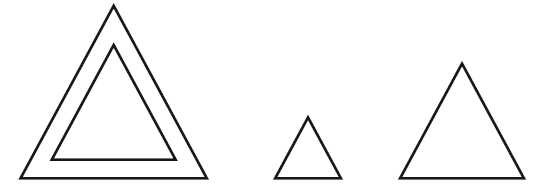Also, Two polygons with the same number of sides are similar, if

• Their corresponding angles are equal and
• Their corresponding sides are in the same ratio (or proportion).

Remember, The ratio of corresponding sides of a polygon is referred as the scale factor or representative fraction.

## Similar Triangles:

The same rule which is applied on similarity of polygon is done with it.

Two triangles are similar, if

(i) Their corresponding angles are equal and

(ii) Their corresponding sides are in the same ratio (or proportion).

Geometrically:

1. ∠A = ∠D, ∠B = ∠E, ∠C = ∠F
2. $$\frac{AB}{DE}$$ = $$\frac{BC}{EF}$$ = $$\frac{CA}{FD}$$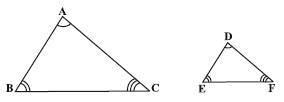Then the two triangles will be similar.

### Basic proportionality Theorem or Thale’s Theorem:

The ratio of any two corresponding sides in two equiangular triangles is always same.

i.e. $$\frac{AD}{DB}$$ = $$\frac{AE}{EC}$$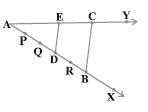## Some properties related to similar triangles:

1. If a line is drawn parallel to one side of a triangle and it intersects the other two sides at distinct points, the other two sides are divided in the same ratio.i.e. $$\frac{AD}{DB}$$ = $$\frac{AE}{EC}$$
2. If a line divides any two sides of a triangle in the same ratio, then the line must be parallel to the third side.
i.e. DE || BC3. If in two triangles, corresponding angles are equal, then their corresponding sides are in the same ratio (or proportion) and hence the two triangles are similar.
4. AA similarity rule: If two angles of one triangle are respectively equal to two angles of another triangle, then the two triangles are similar.
5. SSS similarity rule: If in two triangles, sides of one triangle are proportional to (e., in the same ratio of ) the sides of another triangle, then their corresponding angles must be equal and hence the two triangles are similar.
6. SAS similarity rule: If one angle of a triangle is equal to one angle of the other triangle and the sides including these angles are proportional, then the two triangles are similar.
7. Area Rule: The ratio of the areas of two similar triangles is equal to the square of the ratio of their corresponding sides in every aspect.8. Right Triangle Rule: If in a right triangle, a perpendicular is drawn from the vertex of the right angle to the hypotenuse, then triangles on both sides of the perpendicular are similar to the whole and to each other.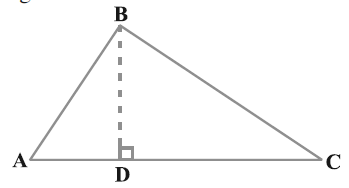9. Pythagorean inverse: In a triangle, if a square of one side is equal to the sum of the squares of the other two sides, then the angle opposite the first side is a right angle.

## Some examples related to similarity of triangle:

1. In the given figure, If PQ || RS, prove that ∆POQ ~ ∆SOR.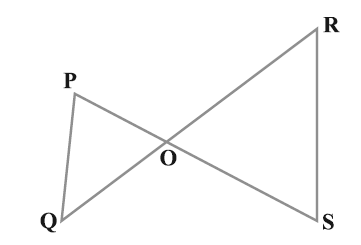Solution:

PQ || RS    (Given)

So, ∠P = ∠S     (Alternate angles)

And, ∠Q = ∠R

∠POQ = ∠SOR     (Vertically opposite angles)

Thus, ∆POQ ~ ∆SOR    (AAA similarity rule)

1.  In the given figure, if OA .OB = OC .OD. Show that A = C and B = ∠D.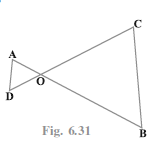Solution:

Given that,

OA .OB = OC .OD

So, $$\frac{OA}{OC}$$ = $$\frac{OD }{OB}$$   …..(i)

Also, ∠AOD = ∠COB   (vertically opposite angles)   …..(ii)

From (i) and (ii)

∆AOD ~ ∆COB …. (SAS similarity rule)

Thus, A = C and B = D.

For further  information please visit Information corner